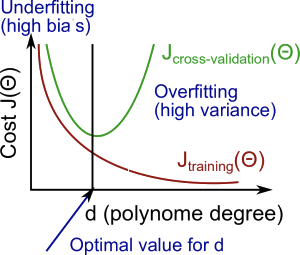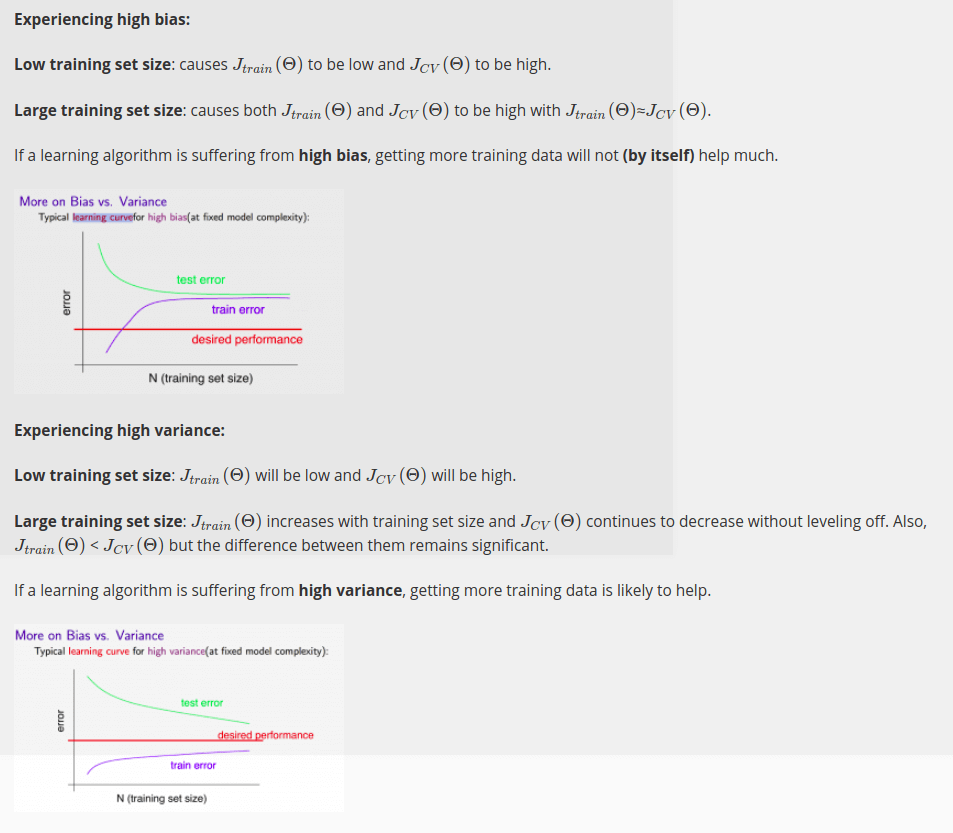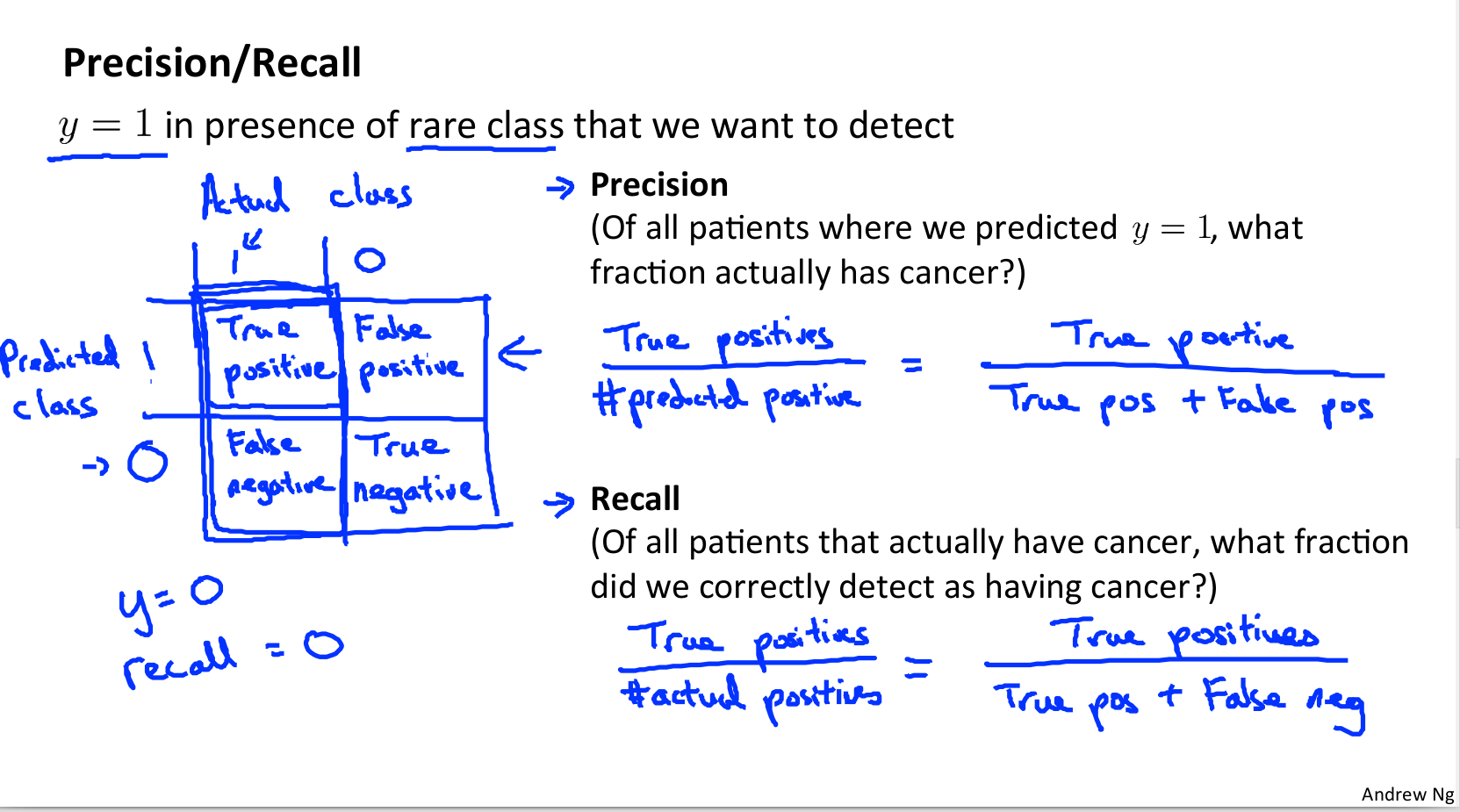# Sail

### 课程部分内容记录

#### Evaluating a Hypothesis

Once we have done some trouble shooting for errors in our predictions by:

1.Getting more training examples
2.Trying smaller sets of features
4.Trying polynomial features
5.Increasing or decreasing λ
6.We can move on to evaluate our new hypothesis.

A hypothesis may have a low error for the training examples but still be inaccurate (because of overfitting). Thus, to evaluate a hypothesis, given a dataset of training examples, we can split up the data into two sets: a training set and a test set. Typically, the training set consists of 70 % of your data and the test set is the remaining 30 %.

#### Diagnosing Bias vs. Variance

High bias (underfitting): both $J_{train}(\Theta)$ and $J_{CV}(\Theta)$ will be high. Also, $J_{CV}(\Theta) \approx J_{train}(\Theta)$
High variance (overfitting): $J_{train}(\Theta)$ will be low and $J_{CV}(\Theta)$ will be much greater than $J_{train}(\Theta)$
The is summarized in the figure below:#### Learning Curves#### Deciding What to Do Next Revisited

Our decision process can be broken down as follows:

1. Getting more training examples: Fixes high variance
2. Trying smaller sets of features: Fixes high variance
3. Adding features: Fixes high bias
4. Adding polynomial features: Fixes high bias
5. Decreasing λ: Fixes high bias
6. Increasing λ: Fixes high variance.